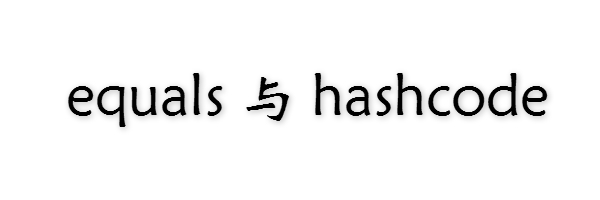## equals 方法

equals 方法来源于 Object 超类；该方法用于检测一个对象与另一个对象是否相等。

### Object 中的 equals

``````public boolean equals(Object obj) {
return (this == obj);
}``````

### 重写 equals

• 自反性

对于对象 x ，`x.equals(x)` 应当始终返回 true。

• 对称性

对于对象 x、y，如果 `x.equals(y)` 返回 true，那么 `y.equals(x)` 也必须返回 true。

• 传递性

对于对象 x、y、z，如果 `x.equals(y)` 返回 true，`y.equals(z)` 返回 true；那么 `x.equals(z)` 也必须返回 true。

• 一致性

对于对象 x、y，如果 `x.equals(y)` 返回 true，那么反复调用的结果应当一直为 true。

• 空值不行等性

对于任意非空对象 x，`x.equals(null)` 应当永远返回 false。

• 对象属性的冲突

### getClass 的使用

``````public boolean equals(Object obj){
// 进行完全匹配检测(引用)
if(this==obj) return true;
// 进行空值检测
if(obj==null) return false;
// 进行类型匹配检测
if(this.getClass()!=obj.getClass()) return false;
// 进行属性相等检测，省略...
}``````

### instanceof 的使用

``````public boolean equals(Object obj){
// 进行完全匹配检测(引用)
if(!(this instaceof obj)) return false;
// 进行空值检测
if(obj==null) return false;
// 进行类型匹配检测
if(this.getClass()!=obj.getClass()) return false;
// 进行属性相等检测，省略...
}``````

### getClass 与 instaceof 的取舍

• 如果子类拥有自己的相等性概念，则对称性强制要求采用 getClass 方式检测。
• 如果由超类决定相等性概念，那么就可以采用 instanceof 检测，保证我们可以在子类对象间进行相等性判断。

### 重写 equals 的建议

• 首先检测 this 与 otherObject 是否引用同一对象
``if(this==otherObject) return true;``
• 然后检测 otherObject是否为 null，如果为 null 返回 false，这是必须的
``if(otherObject == null) return false;``
• 其次比较 this 与 otherObject 是否同属于一个类；如果 equals 语义在子类中有所改变，则 使用 getClass 检测
``if(this.getClass()!=otherObject.getClass()) return false;``
• 最后将 otherObject强制转换为 当前类型，并进行属性值检测；注意：如果在子类中重写的equals，则需要在重写时首先进行 `super.equals(other)` 判断

## hashcode 方法

• hashcode 方法是干啥的？
• hashcode(哈希值) 是个什么玩意？
• hashcode 有什么用？
• 我为啥要重写 hashcode？
• 我不重写它有啥后果？

### hashcode 有什么用？

hashcode(哈希值) 的作用就是用于在使用 Hash算法实现的集合中确定元素位置。

O(1)级别说白了就是 在任意数据大小的容器中，取出一个元素所使用的时间与元素个数无关；通俗的说法就是 不论你这个 HashMap 里有100个元素还是有9999999个元素，我通过 key 取出一个元素所使用的时间是一样的。

### 我为啥要重写 hashcode && 不重写有啥后果

``````public class Pserson {
private String name;
private int age;

// 重写 equals
@Override
public boolean equals(Object obj) {
if (this == obj)
return true;
if (obj == null)
return false;
if (getClass() != obj.getClass())
return false;

// 主要在这，我们根据业务逻辑，即 姓名和年龄 确立相等关系
pserson other = (pserson) obj;
if (age != other.age)
return false;
if (name == null) {
if (other.name != null)
return false;
} else if (!name.equals(other.name))
return false;
return true;
}
}``````

• hashcode 方法返回对象的 哈希值；
• 我们通过 哈希值 的运算(与指定数取余等)来确立元素在 hash 算法实现的容器中的位置；
• Object 中的 hashcode 方法 对于业务逻辑上相等的两个对象(属性相同，不同引用) 返回的 hashcode 是不同的。

``````public class Test1 {
public static void main(String[] args) {
Pserson pserson1 = new Pserson();
pserson1.setName("张三");
pserson1.setAge(10);

Pserson pserson2 = new Pserson();
pserson2.setName("张三");
pserson2.setAge(10);

HashSet<Pserson> hashSet = new HashSet<Pserson>();